# NASA and conformal maps

A couple years ago I wrote about how NASA was interested in regions bounded by curves of the formFor example, here’s a plot for A = 2, B = 1, α = 2.5 and β = 6.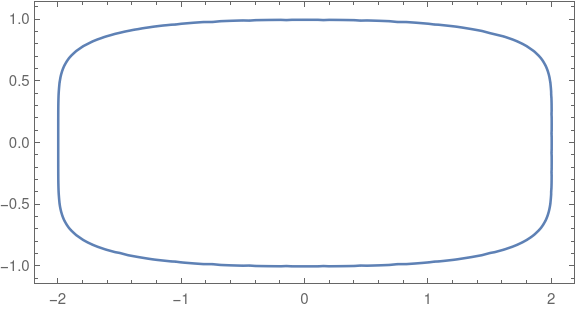That post mentioned a technical report from NASA that explains why these shapes are important in application, say for beams and bulkheads, and gives an algorithm for finding conformal maps of the unit disk to these shapes. These shapes are also used in graphic design, such as squircle buttons on iPhones.

Note that these shapes are not rounded rectangles in the sense of a rectangles modified only at the corners. No segment of the sides is perfectly flat. The curvature smoothly decreases as you move away from the corners rather than abruptly jumping to 0 as it does in a rectangle rounded only at the corners. Maybe you could call these shapes continuously rounded rectangles.

## Conformal maps

Conformal maps are important because they can map simple regions to more complicated regions in way that locally preserves angles. NASA might want to solve a partial differential equation on a shape such as the one above, say to calculate the stress in a cross section of a beam, and use conformal mapping to transfer the equation to a disk where the calculations are easier.

## Coefficients

The NASA report includes a Fortran program that computes the coefficients for a power series representation of the conformal map. All the even coefficients are zero by reasons of symmetry.

Coefficients are reported to four decimal places. The closer the image is to a circle, i.e. the closer α and β are to 2 and the closer A is to B, the fewer non-zero coefficients the transformation has. You could think of the number of non-zero coefficients as a measure how how hard the transformation has to work to transform the disk into the desired region.

There are interesting patterns in the coefficients that I would not have noticed if I had not had to type the coefficients into a script for the example below. Maybe some day I’ll look more into that.

## Conformal map example

The following example uses A = 2, B = 1, and α = β = 5.And here is the image of the disk under the conformal map giving by the coefficients in the NASA report.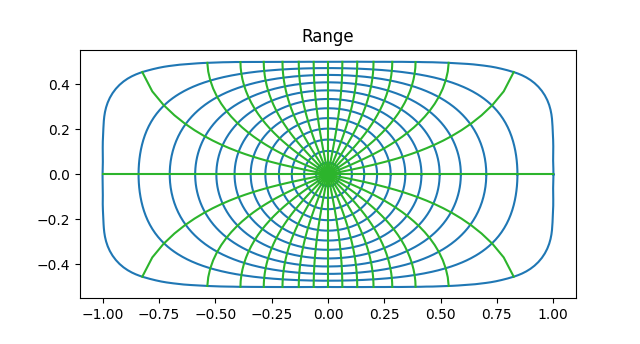Note that the inner most circles from the domain are mapped into near circles, but as you move outward the circles are distorted more, approaching the shape of the boundary of the range.This is because in the limit, analytic functions map disks to disks. So a tiny circle around the origin will always map to an approximate circle, regardless the target region.

Note also that although the circles and lines of the domain are warped in the range, they warp in such a way that they still intersect at right angles. This is the essence of a conformal map.

## Inverse map

The NASA report only gives a way to compute the transformation from the disk to the target region. Typically you need to be able to move both ways, disk to target and target to disk. However, since the transformation is given by a power series, computing the inverse transformation is a problem that has been solved in general. This post gives an explanation and an example.

# Transformations of Olympic rings

The previous post gave the details of how Möbius transformations

m(z) = (az + b)/(cz + d)

transform circles. The image of a circle under a Möbius transformation is either a line or a circle, and in our examples the image will always be a circle.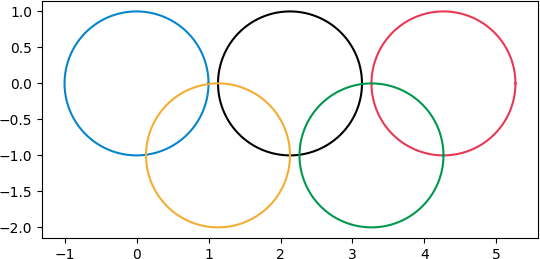and then apply various transformations.

First we apply (z + 9)/(z + 10):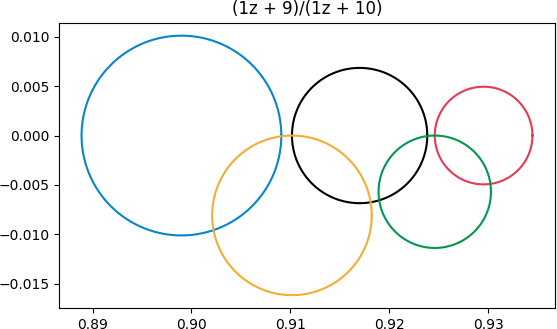This transformation doesn’t distort the rings too much because the rings are far from the singularity at z = -10. The blue ring is distorted the most because it’s closest to the singularity.

Next we apply (iz + 7)/(z + 8).  Now we’ve moved the singularity a little bit closer, and we caused some rotation by using i as the coefficient of z in the numerator.Finally, let’s pick a transformation with its singularity inside the green circle, (z + 4)/(z -3 + i).Now we see a lot of distortion. The blue and gold rings that were outside the green ring are brought inside. The complex plane is turned inside-out because of the singularity.

As before, the black and red rings still intersect the green ring. But what you can’t tell from the graph is that the portion of these rings that was inside has been turned outside and vice versa.

# Circles and lines under a Möbius transformation

This post will revisit a previous post in more detail. I’ve written before about how Möbius transformations map circles and lines to circles and lines. In this post I’d like to explore how you’d calculate which circle or line a given circle or line goes to. Given an equation of a line or circle, what is the equation of its image?

## Circles to circles

A while back I wrote a post called Circles to Circles, demonstrating how Möbius transformations map circles and lines to circles and lines. If you think of a line as a degenerate circle, a circle of infinite radius, then you could simply say Möbius transformations map circles to circles.

For example I showed in that post that the transformation

m(z) = (z + 2)/(3z + 4)

maps these circlesto these circles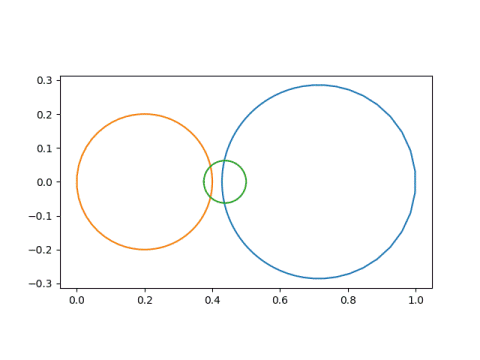and that these lines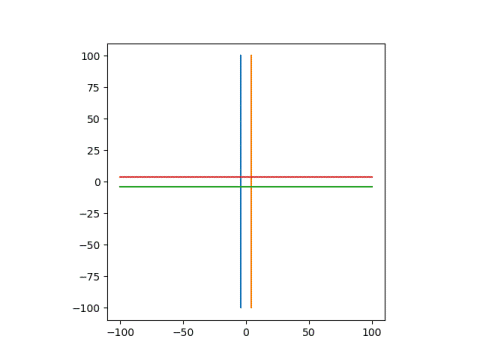go to these circles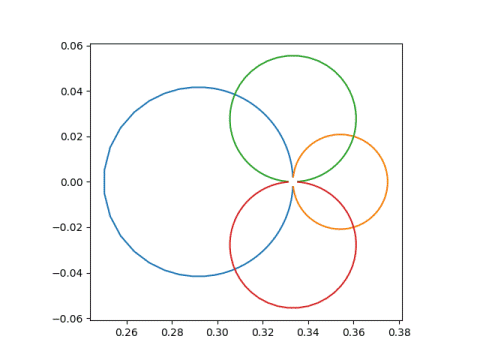## Circle or line image

Given the equation of a circle or a line, how would you know whether its image is a circle or a line?

A Möbius transformation

m(z) = (az + b) / (cz + d)

has a singularity at z = –d/c. If a line or curve goes through the point –d/c then its image is a line. Otherwise the image is a circle.

If the line or curve does go through –d/c, so that the image is a line, it’s easy to find an equation of the line. Pick two points, z1 and z2 on the original curve or line. Let w1m(z1) and w2m(z2). Then find the equation of a line through w1 and w2. For example, a parametric form is

w1 + t(w2w1)

for all real t.

## Equation of the image circle

m(z) = (az + b) / (cz + d)

as above, but we assume our circle does not pass through –d/c since that case is covered above. With this assumption we can conclude that the image of our circle is another circle, and the remaining task is to find an equation of that circle.

We can decompose our transformation m into several simpler steps—translation, reciprocal, rotation, and dilation—and see what happens to the center and radius under each step. The hardest step is taking the reciprocal, and this is covered in the previous post. That post was a lemma for this post.

We can apply m to z in four steps:

2. Take the reciprocal.
3. Multiply by e = (bcad)/c².

We know it’s OK to take the reciprocal in the second step because our circle does not contain the point z = –d/c.

We can break step 3 into (3a) multiplying by |e| and (3b) multiplying by e/|e|. Step 3a is a dilation and step 3b is a rotation.

Here’s a Python program using the steps above to find the center and radius of a transformed circle.

    def transform(a, b, c, d, center, radius):
# step 1
center += d/c

# step 2
temp = center
center = np.conj(center) / (abs(center)**2 - radius**2)

# step 3
e = (b*c - a*d)/c**2
center *= e

# step 4
center += a/c



# Reciprocal of a circle

Let C be a circle in the complex plane with center c and radius r. Assume C does not pass through the origin.

Let f(z) = 1/z. Then f(C) is also a circle. We will derive the center and radius of f(C) in this post.

***

Our circle C is the set of points z satisfyingDefine w = 1/z and substitute 1/w for z above.

A little algebra showsand a little more showsThis is the equation of a circle with centerAs a way to check the derivation above, here’s some Python code for making circles and taking their reciprocal.

    import numpy as np
import matplotlib.pyplot as plt

theta = np.linspace(0, 2*np.pi, 1000)

# plot image of circle of radius r centered at c
def plot(c, r):
cc = np.conj(c)
d = r**2 - c*cc
print("Expected center: ", -cc/d)
u = np.exp(1j * theta) # unit circle
w = 1/(r*u + c)
plt.plot(w.real, w.imag)
plt.gca().set_aspect("equal")
plt.show()

plot(1 + 2j, 3)
plot(0.5, 0.2)
plot(1j, 0.5)


# Computing zeta at even numbers

Last year I wrote several posts about computing ζ(3) where ζ is the Riemann zeta function. For example, this post.

It happens that ζ can be evaluated in closed form at positive even arguments, but there’s still a lot of mystery about zeta at positive odd arguments.

There’s a way to derive ζ(2n) using contour integration. But you don’t need to be clever about constructing contours if you start from the following result which is derived using contour integration.

Suppose f is analytic except for poles at zk and that the sumconverges, where the sum is over all integer n except poles of f. Then the sum can be computed from residues at the poles:Here Res stands for “residue.” The residue of a function g(z) at z0 is defined as the coefficient c-1 of (zz0)-1 in the Laurent series for g. Equivalently,This means we can evaluate infinite sums by taking a limit that may be a simple application of L’Hôpital’s rule.

The theorem above could be used to calculate a lot more than values of the Riemann zeta function by choosing various functions f, but for our purposes f(z) will be z-2m for some positive integer m.Why can’t this same trick be used for evaluating the Riemann zeta function at odd integer arguments? The second equality above fails. For even powers of n, the sum over the positive integers is half the sum over all integers. For odd powers, the sum over all integers is zero.

If we write out the Laurent series for π cot πz then we can read off the residues of π cot πz/z2m.When we divide by z² the coefficient of z becomes the coefficient of z-1, i.e. the residue. This residue is -π²/3, and so negative one half of this is π²/6, i.e. ζ(2) = π²/6.

The same line of reasoning shows that ζ(4) = π4/90 and ζ(6) = π6/945.

Finding the values of ζ(2m) in general is no harder than finding the power series for cotangent, which is not trivial but not insurmountable either. See this post.

The final result is that# No analytic bump

The word “smooth” in mathematics usually means infinitely differentiable. Occasionally the word is used to mean a function has as many derivatives as necessary, but without being specific about how many derivatives that is.

A function is analytic if it has a convergent power series representation at every point of its domain. An analytic function is infinitely differentiable, but surprisingly the converse is not true.

Suppose we took a bump function as described in the previous post, say over the interval [-1, 1]. Such a function would be infinitely differentiable at 1, positive to the left of 1 and zero to the right. Let’s compute the power series centered at 1. All the derivatives are zero at 1, so all the power series coefficients are 0, so the power series sums to 0. The bump function is positive on the left side of 1 but the power series is not.

When does the power series of an infinitely differentiable function converge to that function? More on this subtle question here.

Now suppose we forge ahead and try to construct an analytic bump function. What goes wrong? Do we get something that is almost analytic?

Let’s follow the construction of the previous post, starting with

f(x) = exp(x).

Then

g(x) = 1 /f(1/x) = exp(-1/x)

and

h(x) = g(1+x) g(1-x) = exp(1/(1 – x²))

In Mathematica,

    h[x_] := If[Abs[x] < 1, Exp[-1/(1 - x^2)], 0]

Now let’s look at h from several perspectives. Along the real axis, everything is fine.

    Plot[h[x], {x, -1.1, 1.1}]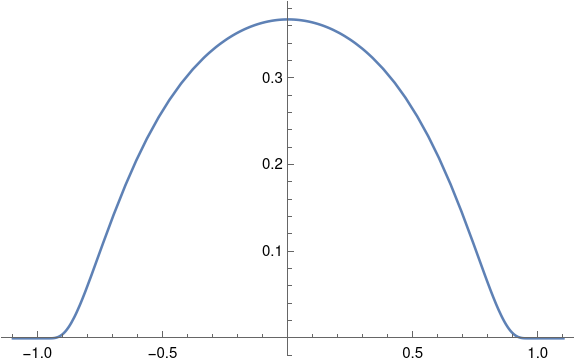The function seems to drop to zero abruptly so that it might not be smooth, but zooming in a little reassures us that the function is indeed smooth.

    Plot[h[x], {x, -1.1, -0.9}, PlotRange -> All]What if we plot h as a complex function?Now things don’t look so smooth. The swirls of color near -1 and 1 show that the phase is changing violently. Also, the light color in the unit circle compared to the dark color outside suggests the phase jumps when we cross the unit circle rather than smoothly transitioning to zero.

Let’s see what happens to the magnitude of h as we cut across the square above diagonally.

    Plot[Abs[h[z I + z]], {z, -1, 1}]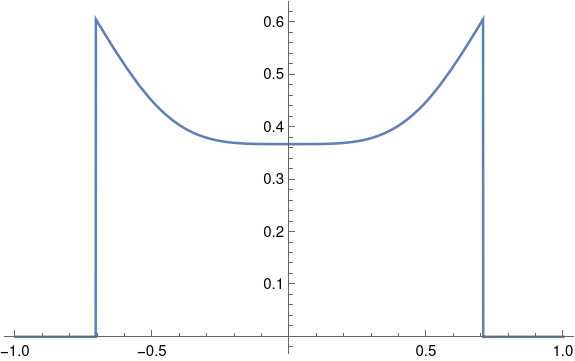Definitely not smooth. Not even continuous.

Let’s see what happens if we add a dimension to our plot, using |h(z)| as height.

    ComplexPlot3D[h[z], {z, -1.1 - 1.1 I, 1.1 + 1.1 I}]So a lot is happening at ±1. Let’s turn this plot around to look at it from a different perspective.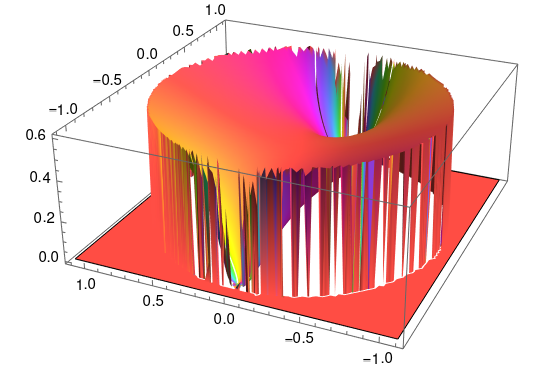Our function has essential singularities at ±1 when we look at it in the complex plane. Along the real axis everything looks fine, but when we look at it from the complex plane we see that the function is very badly behaved.

Before we go, let’s go back and look at the example from the previous post, a bump function that is six derivatives along the real axis. What would it look like extended to the complex plane?

    h2[z_] := If[Abs[z] < 1, (1 + z)^7 (1 - z)^7, 0]
ComplexPlot3D[h2[z], {z, -1.1 - 1.1 I, 1.1 + 1.1 I}]


This gives us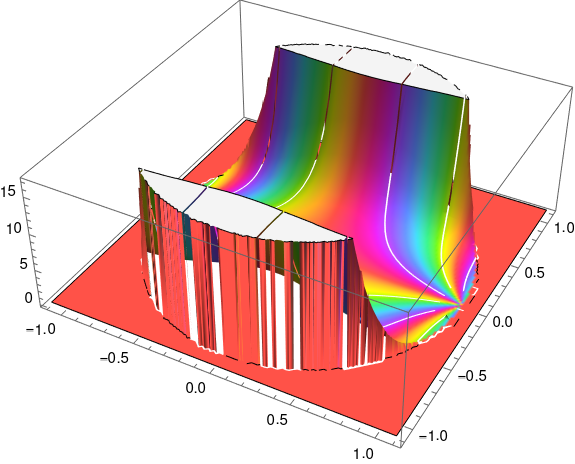Here's the same plot viewed from a different angle.# Bump functions

A bump function is a smooth (i.e. infinitely differentiable) function that is positive on some open interval (a, b) and zero outside that interval. I mentioned bump functions a few weeks ago and discussed how they could be used to prevent clicks in radio transmissions.

Today I ran into a twitter thread that gave a more general construction of bump functions that I’d seen before. The thread concludes with this:

You can create bump functions by this recipe:

1. Take any f(x) growing faster than polynomial (e.g. exp)
2. Define g(x) = 1 / f(1/x)
3. Let h(x) = g(1+x) g(1−x).
4. Zero out x∉(−1,1)
5. Scale, shift, etc

In this post I’ll give a quick asymptotic proof that the construction above works.

Let a positive integer n be given and define g(x) to be zero for negative x. We’ll show that if f grows faster than xn. then g is n times differentiable at 0.

As x → ∞, f(x) is eventually bounded below by a function growing faster than xn. And so as x → 0, f(1/x) grows faster than xn and 1/f(1/x) goes to zero faster than xn, and so its nth derivative is zero.

It follows that g(1 + x) is n times differentiable at -1 and g(1 – x) is n times differentiable at 1. So h is n times differentiable at -1 and 1. The function h is positive on the open interval (-1, 1)  and zero outside. Our choice of n was arbitrary, so h is infinitely differentiable, and so h is a bump function. We could shift and scale h to make it a bump function on any other finite interval.

## Discussion

When I was a student, I would have called this kind of proof hand waving. I’d want to see every inequality made explicit: there exists some M > 0 such that for x > M …. Now I find arguments like the one above easier to follow and more convincing. I imagine if a lecturer gives a proof with all the inequalities spelled out, he or she is probably thinking about something like the proof above and expanding the asymptotic argument on the fly.

Note that a slightly more general theorem falls out of the proof. Our goal was to show if f grows faster than every polynomial, then g and h are infinitely differentiable. But along the way we proved, for example, that if f eventually grows like x7 then g and h are six-times differentiable.

In fact, let’s look at the case f(x) = x7.

    f[x_] := x^7
g[x_] := If[x > 0, 1/ f[1/x], 0]
h[x_] := g[x + 1] g[1 - x]
Plot[h[x], {x, -1.5, 1.5}]


This produces the following plot.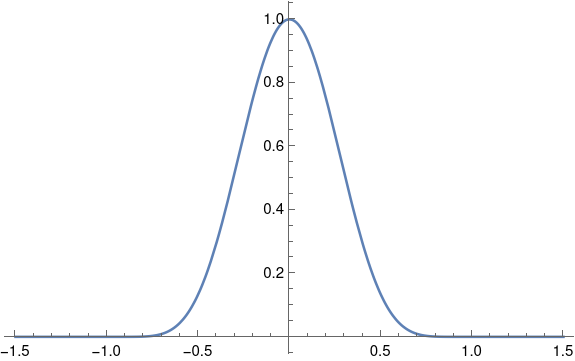Just looking at the plot, h looks smooth; it’s plausible that it has six derivatives. It appears h is zero outside [-1, 1] and h is positive over at least part of [-1, 1]. If you look at the Mathematica code you can convince yourself that h really is positive on the entire interval open interval (-1, 1), though it is very small as it approaches either end.

# The quality of an RNG depends on the application

A random number generator can be good for some purposes and not for others. This isn’t surprising given the fundamentally impossible task such generators are supposed to perform. Technically a random number generator is a pseudo random number generator because it cannot produce random numbers. But random is as random does, and for many purposes the output of a pseudorandom number generator is random for practical purposes. But this brings us back to purposes.

Let β be an irrational number and consider the sequence

xnnβ mod 1

for n = 0, 1, 2, … That is, we start at 0 and repeatedly add β, taking the fractional part each time. This gives us a sequence of points in the unit interval. If you think of bending the interval into a circle by joining the 1 end to 0, then our sequence goes around the circle, each time moving β/360°.

Is this a good random number generator? For some purposes yes. For others no. We’ll give an example of each.

## Integration

If your purpose is Monte Carlo integration, then yes it is. Our sequence has low discrepancy. You can approximate the integral of a function f over [0, 1] by taking the average of f(xn) over the first N elements of the sequence. Doing Monte Carlo integration with this particular RNG amounts to quasi Monte Carlo (QMC) integration, which is often more efficient than Monte Carlo integration.

Here’s an example using β = e.

    import numpy as np

# Integrate f with N steps of (quasi) Monte Carlo
def f(x): return 1 + np.sin(2*np.pi*x)
N = 1000

# Quasi Monte Carlo
sum = 0
x = 0
e = np.exp(1)
for n in range(N):
sum += f(x)
x = (x + e) % 1
print(sum/N)

# Monte Carlo
sum = 0
np.random.seed(20220623)
for _ in range(N):
sum += f(np.random.random())
print(sum/N)


This code prints

    0.99901...
0.99568...


The exact value of the integral is 1, and so the error using QMC between 4 and 5 times smaller than the error using MC. To put it another way, integration using our simple RNG is much more accurate than using the generally better RNG that NumPy uses.

## Simulation

Now suppose we’re doing some sort of simulation that requires computing the gaps between consecutive random numbers. Let’s look at the set of gaps we get using our simple RNG.

    gapset = set()
x = 0
for _ in range(N):
newx = (x + e) % 1
gap = np.abs(newx - x)
x = newx
print( gapset )


Here we rounded the gaps to 10 decimal places so we don’t have minuscule gaps caused by floating point error.

And here’s our output:

    {0.7182818285, 0.2817181715}

There are only two gap sizes! This is a consequence of the three-gap theorem that Greg Egan mentioned on Twitter this morning. Our situation is a slightly different in that we’re looking at gaps between consecutive terms, not the gaps that the interval is divided into. That’s why we have two gaps rather than three.

If we use NumPy’s random number generator, we get 1000 different gap sizes.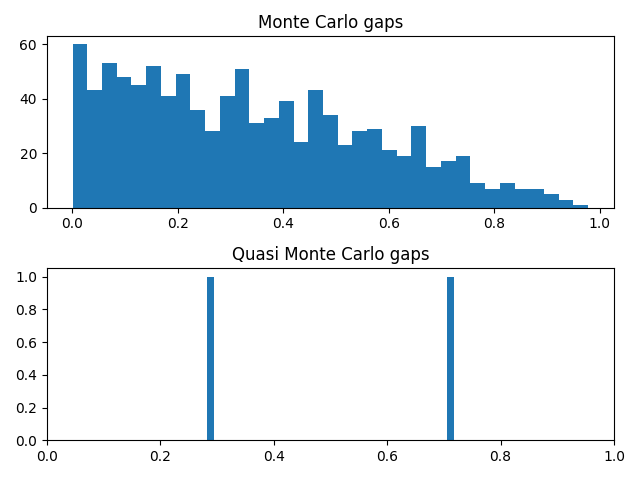## Cryptography

Random number generators with excellent statistical properties may be completely unsuitable for use in cryptography. A lot of people don’t know this or don’t believe it. I’ve seen examples of insecure encryption systems that use random number generators with good statistical properties but bad cryptographic properties.

These systems violate Kirchoff’s principle that the security of an encryption system should reside in the key, not in the algorithm. Kirchoff assumed that encryption algorithms will eventually be known, and difficult to change, and so the strength of the system should rely on the keys it uses. At best these algorithms provide security by obscurity: they’re easily breakable knowing the algorithm, but the algorithm is obscure. But these systems may not even provide security by obscurity because it may be possible to infer the algorithm from the output.

## Fit for purpose

The random number generator in this post would be terrible for encryption because the sequence is trivially predictable. It would also fail some statistical tests, though it would pass others. It passes at least one statistical test, namely using the sequence for accurate Monte Carlo integration.

Even so, the sequence would pass a one-sided test but not a two-sided test. If you tested whether the sequence, when used in Monte Carlo integration, produced results with error below some threshold, it would pass. But if you looked at the distribution of the integration errors, you’d see that they’re smaller than would be expected from a random sequence. The sequence provides suspiciously good integration results, failing a test of randomness but suggesting the sequence might be useful in numerical integration.

# Numerically evaluating a theta function

Theta functions pop up throughout pure and applied mathematics. For example, they’re common in analytic number theory, and they’re solutions to the heat equation.

Theta functions are analogous in some ways to trigonometric functions, and like trigonometric functions they satisfy a lot of identities. This post will comment briefly on an identity that makes a particular theta function, θ3, easy to compute numerically. And because there are identities relating the various theta functions, a method for computing θ3 can be used to compute other theta functions.

The function θ3 is defined bywhereHere’s a plot of θ3 with t = 1 + i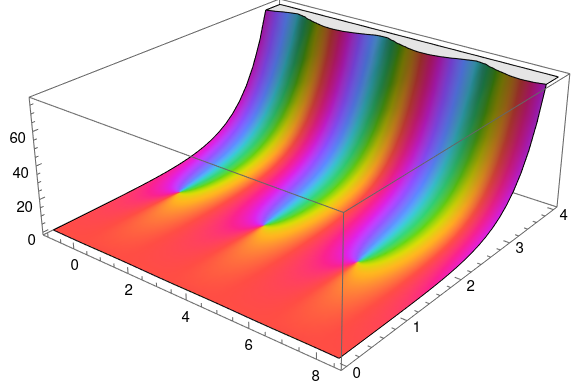produced by the following Mathematica code:


q = Exp[Pi I (1 + I)]
ComplexPlot3D[EllipticTheta[3, z, q], {z, -1, 8.5 + 4 I}]


An important theorem tells usThis theorem has a lot of interesting consequences, but for our purposes note that the identity has the formThe important thing for numerical purposes is that t is on one side and 1/t is on the other.

Suppose t = a + bi with a > 0 and b > 0. ThenNow if b is small, |q| is near 1, and the series defining θ3 converges slowly. But if b is large, |q| is very small, and the series converges rapidly.

So if b is large, use the left side of the theorem above to compute the right. But if b is small, use the right side to compute the left.

# Doubly periodic but not analytic

A sine wave is the canonical periodic function, so an obvious way to create a periodic function of two variables would be to multiply two sine waves:

f(x, y) = sin(x) sin(y)

This function is doubly periodic: periodic in the horizontal and vertical directions.

Now suppose you want to construct a doubly periodic function of a complex variable z = x + iy. One thing you might try is

f(x + iy) = sin(x) sin(y)

This is a function of a complex variable, technically, but it’s not what we usually have in mind when we speak of functions of a complex variable. We’re usually interested in complex functions that are differentiable as complex functions.

If h is the increment in the definition of a derivative, we require the limit as h approaches 0 to be equal no matter what route h takes. On the real line, h could go to zero from the left or from the right. That’s all the possibilities. But in the complex plane, h could approach the origin from any angle, or it could take a more complex route such as spiraling toward 0.

It turns out that it is sufficient that the limit be the same whether h goes to 0 along the real or imaginary axis. This gives us the Cauchy-Riemann equations. If

f(x, y) = u(x, y) + i v(x, y)

then the Cauchy-Riemann equations require the partial derivative of u with respect to x to equal the partial derivative of v with respect to y, and the partial derivative of v with respect to x to be the negative of the partial of u with respect to y.

ux = vy
vx = –uy

These equations imply that if v = 0 then u is constant. A real-valued function of a complex variable cannot be analytic unless its constant.

So can we rescue our example by making up an imaginary component v? If so

f(x, y) = sin(x) sin(y) + i v(x, y)

would have to satisfy the Cauchy-Riemann equations. The first equation would require

v(x, y) = – cos(x) cos(y) + a cos(x)

for some constant a, but the second would require

v(x, y) =  cos(x) cos(y) + cos(y)

for some constant b. Note the negative sign in the former but not in the latter. I’ll leave it as an exercise for the reader to show that these requirements are contradictory.

Liouville’s theorem says that a bounded analytic function must be constant. If an analytic function f is doubly periodic, then it must either be constant or have a singularity. There are many such functions, known as elliptic functions.